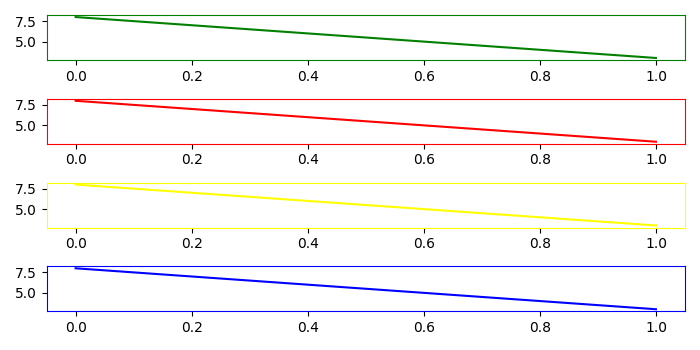# How to change the color of a plot frame in Matplotlib?

MatplotlibServer Side ProgrammingProgramming

To change the color of a plot frame, we can set axes ticklines and spine value into a specific color.

## Steps

• Create a figure and add a set of subplots, using subplots method with value 4.

• Zip colors with axes and iterate them together.

• In the iteration, set the color for spines values and ticklines (x, y).

• To display the figure, use show() method.

## Example

import matplotlib.pyplot as plt
plt.rcParams["figure.figsize"] = [7.00, 3.50]
plt.rcParams["figure.autolayout"] = True
fig, (ax1, ax2, ax3, ax4) = plt.subplots(4)
for ax, color in zip([ax1, ax2, ax3, ax4], ['green', 'red', 'yellow', 'blue']):
plt.setp(ax.spines.values(), color=color)
ax.plot([8, 3], c=color)
plt.setp([ax.get_xticklines(), ax.get_yticklines()], color=color)
plt.show()

## Output